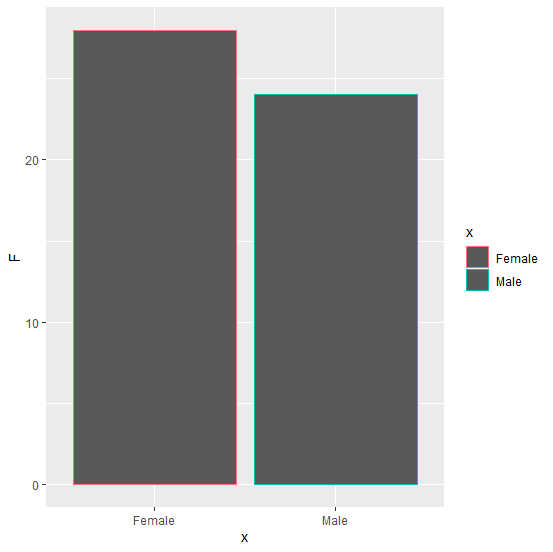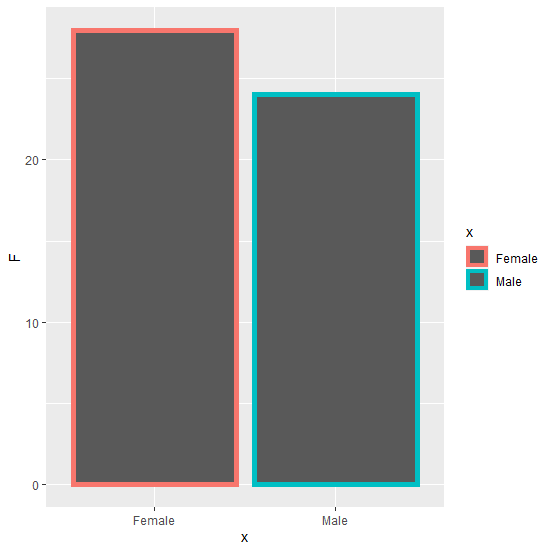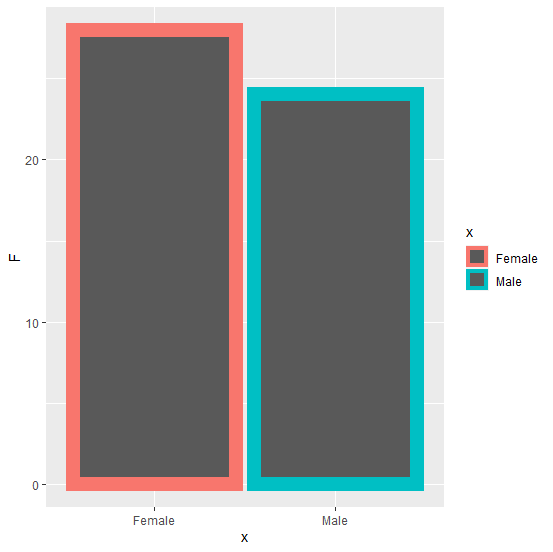# How to change the thickness of the borders of bars in bar plot created by using ggplot2 in R?

R ProgrammingServer Side ProgrammingProgramming

The border thickness highlights the bars and this could be useful in situations where we have similar frequencies. If we want to change the thickness of the bars then size argument under geom_bar function of ggplot2 package can be used and it can be set according to our need starting from 1.

## Example

Consider the below data frame −

x<−c("Male","Female")
F<−c(24,28)
df<−data.frame(x,F)
df

## Output

   x    F
1 Male 24
2 Female 28

library(ggplot2)
ggplot(df,aes(x,F,color=x))+geom_bar(stat="identity")

## OutputCreating the same plot with a little bit larger border size −

ggplot(df,aes(x,F,color=x))+geom_bar(stat="identity",size=2)

## OutputCreating the same plot with a very large border size −

ggplot(df,aes(x,F,color=x))+geom_bar(stat="identity",size=5)

## Output